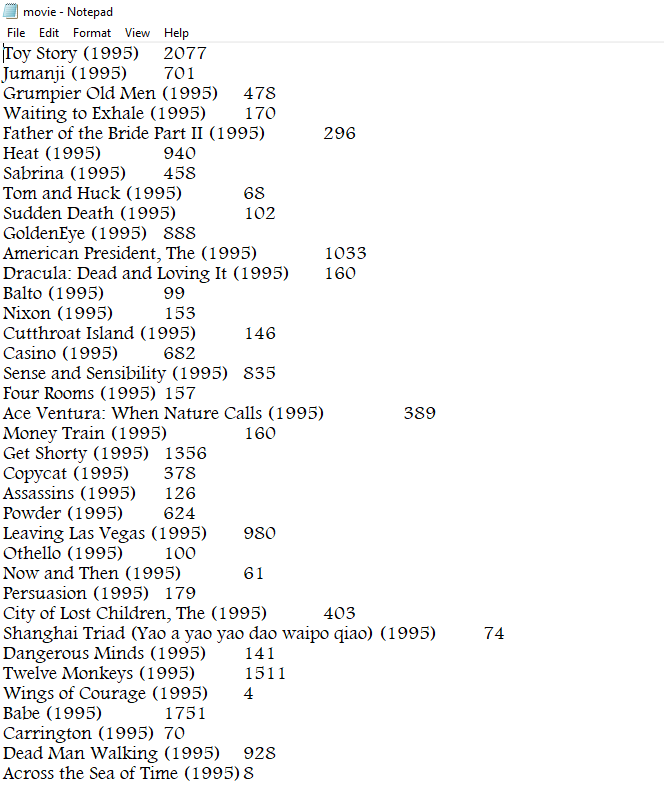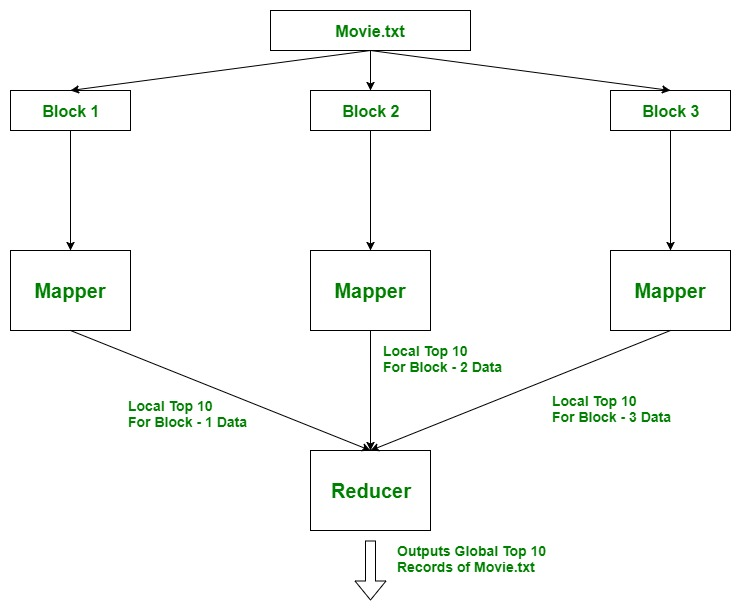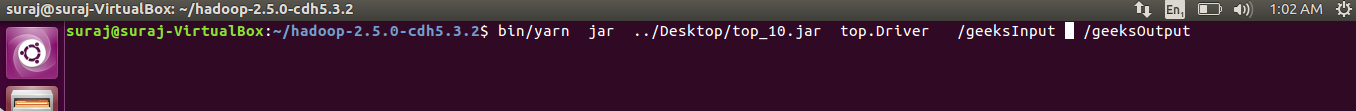Open in App
Not now

# How to find top-N records using MapReduce

• Last Updated : 14 Dec, 2022

Finding top 10 or 20 records from a large dataset is the heart of many recommendation systems and it is also an important attribute for data analysis. Here, we will discuss the two methods to find top-N records as follows.

Method 1: First, let’s find out top-10 most viewed movies to understand the methods and then we will generalize it for ‘n’ records.

Data format:

`movie_name and no_of_views (tab separated)`

Approach Used: Using TreeMap. Here, the idea is to use Mappers to find local top 10 records, as there can be many Mappers running parallelly on different blocks of data of a file. And then all these local top 10 records will be aggregated at Reducer where we find top 10 global records for the file.

Notice: This approach only work if we assume that 2 movie can  not have the same number of views. Otherwise, only one of those two movie will be returned.

Example: Assume that file(30 TB) is divided into 3 blocks of 10 TB each and each block is processed by a Mapper parallelly so we find top 10 records (local) for that block. Then this data moves to the reducer where we find the actual top 10 records from the file movie.txtMapper code:

## Java

 `import` `java.io.*;` `import` `java.util.*;` `import` `org.apache.hadoop.io.LongWritable;` `import` `org.apache.hadoop.io.Text;` `import` `org.apache.hadoop.mapreduce.Mapper;`   `public` `class` `top_10_Movies_Mapper` `    ``extends` `Mapper {`   `    ``private` `TreeMap tmap;`   `    ``@Override` `    ``public` `void` `setup(Context context)` `        ``throws` `IOException, InterruptedException` `    ``{` `        ``tmap = ``new` `TreeMap();` `    ``}`   `    ``@Override` `    ``public` `void` `map(Object key, Text value, Context context)` `        ``throws` `IOException, InterruptedException` `    ``{`   `        ``// input data format => movie_name` `        ``// no_of_views (tab separated)` `        ``// we split the input data` `        ``String[] tokens = value.toString().split(``"\t"``);`   `        ``String movie_name = tokens[``0``];` `        ``long` `no_of_views = Long.parseLong(tokens[``1``]);`   `        ``// insert data into treeMap,` `        ``// we want top 10 viewed movies` `        ``// so we pass no_of_views as key` `        ``tmap.put(no_of_views, movie_name);`   `        ``// we remove the first key-value` `        ``// if it's size increases 10` `        ``if` `(tmap.size() > ``10``) {` `            ``tmap.remove(tmap.firstKey());` `        ``}` `    ``}`   `    ``@Override` `    ``public` `void` `cleanup(Context context)` `        ``throws` `IOException, InterruptedException` `    ``{` `        ``for` `(Map.Entry entry :` `             ``tmap.entrySet()) {`   `            ``long` `count = entry.getKey();` `            ``String name = entry.getValue();`   `            ``context.write(``new` `Text(name),` `                          ``new` `LongWritable(count));` `        ``}` `    ``}` `}`

Explanation:

The important point to note here is that we use “context.write()” in cleanup() method which runs only once at the end in the lifetime of Mapper. Mapper processes one key-value pair at a time and writes them as intermediate output on local disk. But we have to process whole block (all key-value pairs) to find top10, before writing the output, hence we use context.write() in cleanup().

Reducer code:

## Java

 `import` `java.io.IOException;` `import` `java.util.Map;` `import` `java.util.TreeMap;` `import` `org.apache.hadoop.io.LongWritable;` `import` `org.apache.hadoop.io.Text;` `import` `org.apache.hadoop.mapreduce.Reducer;`   `public` `class` `top_10_Movies_Reducer` `    ``extends` `Reducer {`   `    ``private` `TreeMap tmap2;`   `    ``@Override` `    ``public` `void` `setup(Context context)` `        ``throws` `IOException, InterruptedException` `    ``{` `        ``tmap2 = ``new` `TreeMap();` `    ``}`   `    ``@Override` `    ``public` `void` `reduce(Text key,` `                       ``Iterable values,` `                       ``Context context)` `        ``throws` `IOException, InterruptedException` `    ``{`   `        ``// input data from mapper` `        ``// key             values` `        ``// movie_name         [ count ]` `        ``String name = key.toString();` `        ``long` `count = ``0``;`   `        ``for` `(LongWritable val : values) {` `            ``count = val.get();` `        ``}`   `        ``// insert data into treeMap,` `        ``// we want top 10 viewed movies` `        ``// so we pass count as key` `        ``tmap2.put(count, name);`   `        ``// we remove the first key-value` `        ``// if it's size increases 10` `        ``if` `(tmap2.size() > ``10``) {` `            ``tmap2.remove(tmap2.firstKey());` `        ``}` `    ``}`   `    ``@Override` `    ``public` `void` `cleanup(Context context)` `        ``throws` `IOException, InterruptedException` `    ``{`   `        ``for` `(Map.Entry entry :` `             ``tmap2.entrySet()) {`   `            ``long` `count = entry.getKey();` `            ``String name = entry.getValue();` `            ``context.write(``new` `LongWritable(count),` `                          ``new` `Text(name));` `        ``}` `    ``}` `}`

Explanation: Same logic as mapper. Reducer processes one key-value pair at a time and writes them as final output on HDFS. But we have to process all key-value pairs to find top10, before writing the output, hence we use cleanup().

## Java

 `import` `org.apache.hadoop.conf.Configuration;` `import` `org.apache.hadoop.fs.Path;` `import` `org.apache.hadoop.io.LongWritable;` `import` `org.apache.hadoop.io.Text;` `import` `org.apache.hadoop.mapreduce.Job;` `import` `org.apache.hadoop.mapreduce.lib.input.FileInputFormat;` `import` `org.apache.hadoop.mapreduce.lib.output.FileOutputFormat;` `import` `org.apache.hadoop.util.GenericOptionsParser;`   `public` `class` `Driver {`   `    ``public` `static` `void` `main(String[] args) ``throws` `Exception` `    ``{` `        ``Configuration conf = ``new` `Configuration();` `        ``String[] otherArgs` `            ``= ``new` `GenericOptionsParser(conf, args)` `                  ``.getRemainingArgs();`   `        ``// if less than two paths` `        ``// provided will show error` `        ``if` `(otherArgs.length & lt; ``2``) {` `            ``System.err.println(" Error` `                               ``: please provide two paths` `                                     ``& quot;);` `            ``System.exit(``2``);` `        ``}`   `        ``Job job` `            ``= Job.getInstance(conf, " top ``10` `& quot;);` `        ``job.setJarByClass(Driver.``class``);`   `        ``job.setMapperClass(top_10_Movies_Mapper.``class``);` `        ``job.setReducerClass(top_10_Movies_Reducer.``class``);`   `        ``job.setMapOutputKeyClass(Text.``class``);` `        ``job.setMapOutputValueClass(LongWritable.``class``);`   `        ``job.setOutputKeyClass(LongWritable.``class``);` `        ``job.setOutputValueClass(Text.``class``);`   `        ``FileInputFormat.addInputPath(` `            ``job, ``new` `Path(otherArgs[``0``]));` `        ``FileOutputFormat.setOutputPath(` `            ``job, ``new` `Path(otherArgs[``1``]));`   `        ``System.exit(job.waitForCompletion(``true``) ? ``0` `: ``1``);` `    ``}` `}`

Running the jar file:

• We export all the classes as jar files.
• We move our file movie.txt from local file system to /geeksInput in HDFS.
`bin/hdfs dfs -put ../Desktop/movie.txt  /geeksInput`
• We now run the yarn services to run the jar file.
`bin/yarn  jar  jar_file_location  package_Name.Driver_classname   input_path  output_path `• We make our custom parameter using set() method.
`configuration_object.set(String name, String value) `
• This value can be accessed in any Mapper/Reducer by using get() method

```Configuration conf = context.getConfiguration();

// we will store value in String variable
String  value = conf.get(String name);                 ```
•

My Personal Notes arrow_drop_up
Related Articles